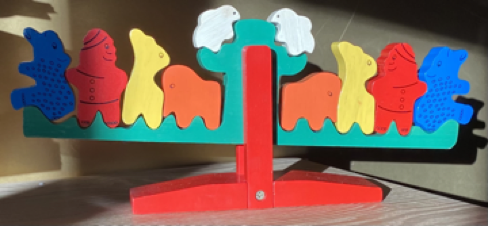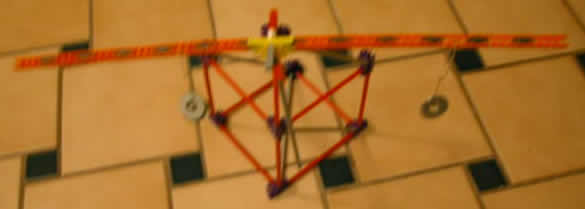# Equality & the = symbol and the equal arm balance

## Introduction

Equality is a major relationship in mathematics. This page discusses it, how learners develop its understanding and use in mathematics with activities to facilitate its development.

## Big idea

Equality or mathematical equivalence is a relationship between quantities that are equal and interchangeable. It is represented by the mathematical symbol =, which is preferably read as, is the same amount as, rather than equal. Or defining equal as having infinite values, find some. Which, would require not having one value as correct, but unlimited values with some being more desirable.

Supporting concepts:

• Any value or amount can be represented in infinite ways.
• Any amount or value can be represented in an algebraic equation or expression.
• Any expression or equation can be represented in infinite ways.

## Development

Young learners see elements and sets as individual and mostly unrelated. The idea of alike, similar, same, twin are recognized as similar, but different. Followed by relationships as being identical and equal; and even later interchangeable based on transitive reasoning.

The idea of two different things being equal and interchangeable, as having no difference, is hard for learners to believe and accept. It seems humans are always looking at things, that occupy different spaces, as being different. When they are told they are the same or interchangeable, they look for something, no matter how small, to be different. To rationalize the separation.

Similarly when they see two different things in two different positions, they consider them different and it is hard for them to consider them as equal or interchangeable.

When learning to understand mathematical relationships and how to communicate them with mathematical statements it takes many experiences to become literate with the idea of interchangeable relationships.

Some common errors include:

• Limiting the term equal as applying to one and only one value.
• Interpret the equals symbol as a symbol for an operation meaning, to find the total or calculate.
• Read the equals symbol as calculate. 2 + 3 + 4 = __ tw0 plus three plus four is ... answer 9.
• Ignoring the equal symbol and add all the values. 2 + 3 = 4 + _ ; responding with 9.

## Scoring guide equality

### Equality & meaning of = symbol (scoring guide & rubric)

Top level

• Equal symbol means there is a relationship between numbers and or expressions represented on either side of the equal sign, which are interchangeable and could be infinite possibilities. A relationship among the numbers or expressions, which can be compared with or without calculations.
• Equal symbol means there is a relationship between two equal numbers that can be found through calculations on each side of the equal symbol.
• Understands that a number sentence doesn't have to be of the form a + b = c.
It might be:
• 5+2=2+5,
• 7=5+2,
• 5+2=7+0,
• ...
• Understands the equal symbol consistently communicates something.
It might be:
• Equal symbol means the answer comes next, or
• Use all the numbers to calculate an answer, or
• Extend the problem put 12 + 5 = 17

Lower level

Related information: Equal arm balance

## Developing fluency with interchangeable values.

• Present equations in different forms to solve and have learners write equations in different ways.

### Different ways to write equations.

Simple equations (notice pattern of change)

• _ = 2 + 3
• 5 = _ + 3
• 5 = 2 + _
• 2 + 3 = _

Complex equations (notice pattern of change)

• _ + 2 = 3 + 4
• 5 + _ = 3 + 4
• 5 + 2 = _ + 4
• 5 + 2 = 3 + _
• 5 + 2 = 3 + 4 = _
• When solving equations with blanks. Ask. What goes in the blank?
• Use the phrase, is the same amount as, instead of equals.
• Use equations instead of number sentences.
• Leave extra space on either side of the = symbol to emphasize it as not and operation.
• Use hand gestures to emphasize each side of an equation. Left hand to draw attention to the left and right hand for the right.
• Use both hands and a balancing motion, as a scale, when referencing same amounts.
• Use is equal to instead of just equals.
• Emphasize, a total doesn't always have to be on either side of an equation.
• Emphasize an equal equation is balanced.

### Activities

#### Sets of similar equations

Have learners create sets of similar equations written in different ways.

• _ = 5 + 2
• 3 + 4 = _
• 1 + 2 + 3 + 1 = _
• 6 + 1/2 + 1/2 = 6 + _
• _ + 2 = 3 + 4
• 5 + _ = 3 + 4
• 5 + 2 = _ + 4
• 5 + 2 = 3 + _
• 5 + 2 = 3 + 4 = _

They can crate cards or pages in a journal for equivalent equations.

Give learners lists of equations to sort into equal to & same as or not equal or same as.

Rearrange equations. Have learners rearrange equations. Challenge them to show ho2 thy are equivalent or not.

Give learners paired equations (equivalent or not) and have them add equivalent, same as, not equivalent, equal, or not equal, and explain their reasoning.

Card games with cards that have different sets to match as equivalent.

## Equal arm balance

An equal arm balance is one of the most important tools for learners to experience for a better understanding of algebra.

A researcher gave a list of mathematical activities to students in algebra classes and had them identified ones they had experienced. Those who checked, experiences with an equal arm balance, was by far the activity with the highest correlation to higher algebra achievement. Followed by and related to understand and use of a variable in multiple roles and understanding the equal sign.

### Toy balance### Equal arm balance (Commercially available)Learners can explore equal arm balances by distributing different weights or masses on each side to discover different combinations that balance.

### Equal arm balance with weights• 4 weights at 2 and
• 1 weight at 8#### Mathematical relationship

In the example above 4 weights at the 2 spot on the left side will balance 1 weight at the 8 spot on the right side.

Learners who discover relationships that balance and generalize a concrete and formal relationship will do better with the study of algebra and equations.

Formally

• weightLeft * distanceLeft = weightRight * distanceRight
• wl * dl = wr * dr

### Equal arm balance with K'Nex®

#### Balance#### Center support#### Balance top view#### Equal arm balance